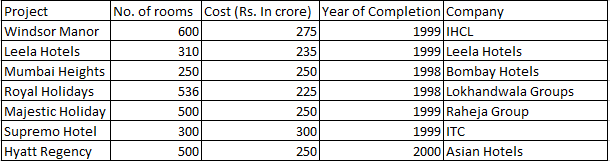### CAT 1997 Question Paper Question 3

Instructions

The following table gives the number of rooms in different hotels in Mumbai and the cost of building each one of them. Note that all the projects started in 1997Note: All projects start in 1997.

Question 3

# Assume that the cost of the project is incurred in the year of completion; interest is charged at the rate of 10% per annum. What is the cost incurred for projects completed in 1998?

Solution

Total cost in 1997 = 250 + 225 = 475 Crores.

These projects were completed in 1998 => Interest for 1 year must be added to the cost.

Interest = $$\frac{475*1*10}{100}$$ = 47.5 Crores

Total Amount = 475 + 47.5 = 522.5 Crores.

• All Quant CAT Formulas and shortcuts PDF
• 30+ CAT previous papers with solutions PDF